## TIFR PHD CS & SS 2020

 Question 1
Two balls are drawn uniformly at random without replacement from a set of five balls numbered 1, 2, 3, 4, 5. What is the expected value of the larger number on the balls drawn?
 A 2.5 B 3 C 3.5 D 4 E None of the above
Engineering-Mathematics       Probability-and-statistics
 Question 2
Let M be a real nXn matrix such that for every non-zero vector xεRn, we have xT Mx > 0. Then
 A Such an M cannot exist. B Such M s exist and their rank is always n. C Such M s exist, but their eigenvalues are always real. D No eigenvalue of any such M can be real. E None of the above.
Engineering-Mathematics       Linear-Algebra
 Question 3A a B b C c D d E e
Engineering-Mathematics       Polynomials-and-vectors
 Question 4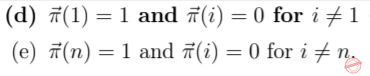A a B b C c D d E e
Engineering-Mathematics       Probability-and-statistics
 Question 5
Let A be an nXn invertible matrix with real entries whose column sums are all equal to 1. Consider the following statements:
(1) Every column in the matrix A2 sums to 2.
(2) Every column in the matrix A3 sums to 3.
(3) Every column in the matrix A-1 sums to 1.
Which of the following is TRUE?
 A none of the statements (1), (2), (3) is correct B statement (1) is correct but not statements (2) or (3) C statement (2) is correct but not statements (1) or (3) D statement (3) is correct but not statements (1) or (2) E all the 3 statements (1), (2), and (3) are correct
Engineering-Mathematics       Linear-Algebra
 Question 6
What is the maximum number of regions that the plane R2 can be partitioned into using 10 lines?
Hint: Let A(n) be the maximum number of partitions that can be made by n lines. Observe that A(0) = 1, A(2) = 2, A(2) = 4 etc. Come up with a recurrence equation for A(n).
 A 25 B 50 C 55 D 56 E 1024
 Question 7
A lottery chooses four random winners. What is the probability that at least three of them are born on the same day of the week? Assume that the pool of candidates is so large that each winner is equally likely to be born on any of the seven days of the week independent of the other winners
 A 17 / 2401 B 48 / 2401 C 105 / 2401 D 175 / 2401 E 294 / 2401
Engineering-Mathematics       Probability-and-statistics
 Question 8
Consider a function f : [0, 1] --> [0, 1] which is twice differentiable in (0, 1). Suppose it has exactly one global maximum and exactly one global minimum inside (0, 1). What can you say about the behaviour of the first derivative f' and and second derivative f'' on (0, 1) (give the most precise answer)?
 A f' is zero at exactly two points, f'' need not be zero anywhere B f' is zero at exactly two points, f'' is zero at exactly one point C f' is zero at at least two points, f'' is zero at exactly one point D f' is zero at at least two points, f'' is zero at at least one point E f' is zero at at least two points, f'' is zero at at least two points
Engineering-Mathematics       Calculus
 Question 9
A contiguous part, i.e., a set of adjacent sheets, is missing from Tharoor’s GRE preparation book. The number on the first missing page is 183, and it is known that the number on the last missing page has the same three digits, but in a different order. Note that every sheet has two pages, one at the front and one at the back. How many pages are missing from Tharoor’s book?
 A 45 B 135 C 136 D 198 E 450
Aptitude       Numerical
 Question 10
In a certain year there were exactly four Fridays and exactly four Mondays in January. On what day of the week did the 20th of January fall that year (recall that January has 31 days)?
 A Sunday B Monday C Wednesday D Friday E None of the others
Aptitude       Numerical
 Question 11
Suppose we toss m = 3 labelled balls into n = 3 numbered bins. Let A be the event that the first bin is empty while B be the event that the second bin is empty. P(A) and P(B) denote their respective probabilities. Which of the following is true?
 A P(A) > P(B) B P(A) = 1/27 C P(A) > P(A|B) D P(A) < P(A|B) E None of the above
Engineering-Mathematics       Probability-and-statistics
 Question 12
The hour needle of a clock is malfunctioning and travels in the anti-clockwise direction, i.e., opposite to the usual direction, at the same speed it would have if it was working correctly. The minute needle is working correctly. Suppose the the two needles show the correct time at 12 noon, thus both needles are together at the 12 mark. After how much time do the two needles meet again?
 A 10/11 hour B 11/12 hour C 12/13 hour D 19/22 hour E One hour
Aptitude       Numerical
 Question 13
What is the area of the largest rectangle that can be inscribed in a circle of radius R?
 A R2/2 B π X R2/2 C R2 D 2R2 E None of the above
Aptitude       Numerical
 Question 14
A ball is thrown directly upwards from the ground at a speed of 10 ms−1, on a planet where the gravitational acceleration is 10 ms−2. Consider the following statements:
1. The ball reaches the ground exactly 2 seconds after it is thrown up
2. The ball travels a total distance of 10 metres before it reaches the ground
3. The velocity of the ball when it hits the ground is 10 ms−1
What can you say now?
 A Only Statement 1 is correct B Only Statement 2 is correct C Only Statement 3 is correct D None of the Statements 1, 2 or 3 is correct E All of the Statements 1, 2 and 3 are correct
Aptitude       Numerical
 Question 15
The sequence s0, s1, . . . , s9 is defined as follows: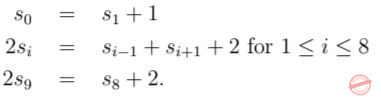what is s0
 A 81 B 95 C 100 D 121 E 190
Aptitude       Numerical
 Question 16A a B b C c D d E None of the above
Digital-Logic-Design       Combinational-Circuit
 Question 17
Consider the following statements.
1. The intersection of two context-free languages is always context-free.
2. The super-set of a context-free language is never regular.
3. The subset of a decidable language is always decidable.
4. Let Σ ≡ {a, b, c} . Let L ⊆ Σ be the language of all strings in which either the number of occurrences of a is the same as the number of occurrences of b OR the number of occurrences of b is the same as the number of occurrences of c. Then, L is not context-free.
Which of the above statements are true?
 A Only (1) B Only (1) and (2) C Only (1),(2) and (3) D Only (4) E None of (1), (2), (3), (4) are true
Theory-of-Computation       Languages-and-Grammars
 Question 18
Consider the (decimal) number 182, whose binary representation is 10110110. How many positive integers are there in the following set?
{n ∈ N : n ≤ 182 and n has exactly four ones in its binary representation}
 A 91 B 70 C 54 D 35 E 27
Digital-Logic-Design       Number-Systems
 Question 19
A clamp gate is an analog gate parametrized by two real numbers a and b, and denoted as clampa,b. It takes as input two non-negative real numbers x and y. Its output is defined asConsider circuits composed only of clamp gates, possibly parametrized by different pairs (a, b) of real numbers. How many clamp gates are needed to construct a circuit that on input non-negative reals x and y outputs the maximum of x and y?
 A 1 B 2 C 3 D 4 E No circuit composed only of clamp gates can compute the max function.
Digital-Logic-Design       Combinational-Circuit
 Question 20A a B b C c D d E e
 Question 21
Consider the context-free grammar below (s denotes the empty string, alphabet is {a, b}):
S → ε|aSb|bSa|SS.
What language does it generate?
 A (ab)∗ + (ba)∗ B (abba)∗ +(baab)∗ C (aabb)∗ + (bbaa)∗ D Strings of the form anbn or bnan, n any positive integer E Strings with equal numbers of a and b
Theory-of-Computation       Languages-and-Grammars
 Question 22
Consider the following algorithm (Note: For positive integers p, q, p/q denotes the floor of the rational number p/q , assume that given p, q, p/q can be computed in one step):
Input: Two positive integers a, b, a ≥ b.
Output: A positive integer g. while (b > 0) {
x = a - (a/b)*b; a = b;
b = x;
}
g = a;
Suppose K is an upper bound on a. How many iterations does the above algorithm take in the worst case?
 A Θ(log K) B Θ(K) C Θ(K log K) D Θ(K2) E Θ(2K)
Algorithms       Time-Complexity
 Question 23
Jobs keep arriving at a processor. A job can have an associated time length as well as a priority tag. New jobs may arrive while some earlier jobs are running. Some jobs may keep running indefinitely. A starvation free job-scheduling policy guarantees that no job waits indefinitely for service. Which of the following job-scheduling policies is starvation free?
 A Round-robin B Shortest job first C Priority queuing D Latest job first E None of the others
Operating-Systems       Process-Scheduling
 Question 24
A particular Panini-Backus-Naur Form definition for a < word > is given by the following rules:Which of the following lexical entities can be derived from < word >?
I. word
II. words
III. c22
 A None of I, II or III B I and II only C I and III only D II and III only E I, II and III
Compiler-Design       Grammars
 Question 25
Among the following asymptotic expressions, which of these functions grows the slowest (as a function of n) asymptotically?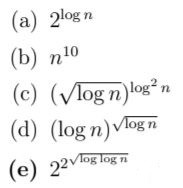A a B b C c D d E e
Algorithms       Asymptotic-Complexity
 Question 26
Which of the following graphs are bipartite?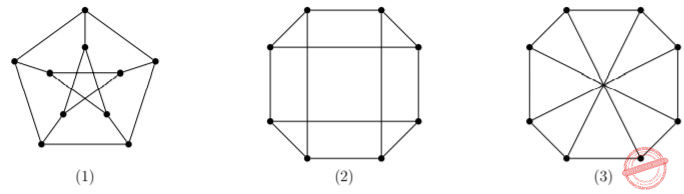A Only (1) B Only (2) C Only (2) and (3) D None of (1),(2), (3) E All of (1), (2), (3)
Engineering-Mathematics       Graph-Theory
 Question 27
Given the pseudocode below for the function remains(), which of the following statements is true about the output, if we pass it a positive integer n > 2?
int remains(int n)
{
int x = n;
for (i=(n-1);i>1;i--) { x = x i;
}
return x;
}
 A Output is always 0 B Output is always 1 C Output is 0 only if n is NOT a prime number D Output is 1 only if n is a prime number E None of the above
Programming       Control-Statement
 Question 28
Let G be an undirected graph. An Eulerian cycle of G is a cycle that traverses each edge of G exactly once. A Hamiltonian cycle of G is a cycle that traverses each vertex of G exactly once. Which of the following must be true?
 A Checking if G has an Eulerian cycle can be done in polynomial time B Deciding if G has a Hamiltonian cycle is not NP-complete C If G has an Eulerian cycle, then it has a Hamiltonian cycle D A complete graph always has both an Eulerian cycle and a Hamiltonian cycle E All of the other statements are true
Engineering-Mathematics       Graph-Theory
 Question 29
The figure below describes the network of streets in a city where Motabhai sells pakoras from his cart. The number next to an edge is the time (in minutes) taken to traverse the corresponding street.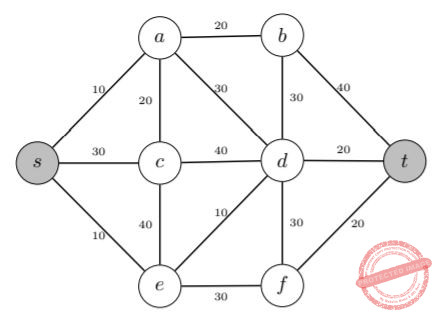At present the cart is required to start at point s and, after visiting each street at least once, reach point t. For example, Motabhai can visit the streets in the following order
s − a − c − s − e − c − d − a − b − d − f − e − d − b − t − f − d − t
in order to go from s to t. Note that the streets b, d and d, f are both visited twice in this strategy. The total time taken for this trip is 440 minutes [which is, 380 (the sum of the traversal times of all streets in the network) + 60 (the sum of the traversal times of streets {b, d} and {d, f })].
Motabhai now wants the cart to return to s at the end of the trip. So the previous strategy is not valid, and he must find a new strategy. How many minutes will Motabhai now take if he uses an optimal strategy?
Hint: s, t, b and f are the only odd degree nodes in the figure above.
 A 430 B 440 C 460 D 470 E 480
 Question 30A 20 B 30 C 32 D 160 E 1024
Digital-Logic-Design       Logic-Gates-and-operators
 Question 31
Consider a discrete-time system which in response to input sequence x[n] (n integer) outputs the sequence y[n] such thatSuppose |α| < 1. Is the system linear, time-invariant, bounded input bounded output (BIBO) stable?
 A Linear, time-invariant, BIBO stable B Non-linear, time-invariant, BIBO stable C Linear, time-variant, BIBO unstable D Non-linear, time-variant, BIBO stable E Cannot be determined from the information given
 Question 32A h(2t) B h(t) C h(t − 1) D h(t + 1) E None of the above
Engineering-Mathematics       Calculus
 Question 33
Balls are drawn one after the other uniformly at random without replacement from a set of eight balls numbered 1, 2, . . . , 8 until all balls drawn. What is the expected number of balls whose value match their ordinality (i.e., their position in the order in which balls were drawn)?
Hint: what is the probability that the i-th ball is drawn at the i-th draw? Now can you use linearity of expectation to solve the problem?
 A 1 B 1.5 C 2 D 2.5 E None of the above
Engineering-Mathematics       Probability-and-statistics
 Question 34
Let f, g : R --> R be two functions that are continuous and differentiable. Consider the following statements:
1. min{f, g} is continuous
2. max{f, g} is continuous
3. max{f, g} is differentiable
Which of the following is TRUE?
 A Only statement 1 is correct B Only statement 2 is correct C Only statement 3 is correct D Only statements 1 and 2 are correct E None of the above
Engineering-Mathematics       Calculus
 Question 35A g(x) is aperiodic B g(x) is periodic with period 1 C g(x) is periodic with period 1 D The value of h determines whether or not g(x) is periodic E None of the above
Engineering-Mathematics       Calculus
 Question 36
For all values of r > 0, the area of the set of all points outside the unit square whose Euclidean distance to the unit square is less than r is: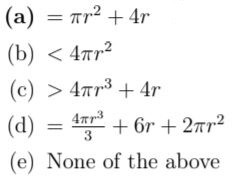A a B b C c D d E e
 Question 37A a B b C c D d E e
Engineering-Mathematics       Probability-and-statistics
 Question 38
Suppose that Dice 1 has five faces numbered 1 to 5, each of which is equally likely to occur once the dice is rolled. Dice 2 similarly has eight equally likely faces numbered 1 to 8. Suppose that the two dice are rolled, and the sum is equal to 8. Conditioned on this, what is the chance that the Dice 1 rolled a number less than or equal to 2?
 A 1/4 B 1/3 C 1/2 D 2/7 E 2/5
Engineering-Mathematics       Probability-and-statistics
 Question 39
Let A be an nXn matrix with the the property that Am = 0 for some mεN. Consider the following statements:
1. At least one entry of A is zero
2. All eigenvalues of A are zero
3. All diagonal entries of A are zero
Which of the following is TRUE?
 A Only statement 1 is correct B Statement 2 alone is correct C Only statement 3 is correct D Only statements 1 and 2 are correct E Only statements 2 and 3 are correct
Engineering-Mathematics       Linear-Algebra
 Question 40
Consider two independent random variables (U1, U2) both are uniformly distributed between [0, 1]. The conditional expectation
E[(U1 + U2)| max(U1, U2) ≥ 0.5]
equals
 A 7/6 B 8/7 C 6/7 D 1.1 E None of the above
Engineering-Mathematics       Probability-and-statistics
 Question 41
Suppose that X is a real valued random variable and E[exp X] = 2. Then, which of the following must be TRUE?
Hint: (exp(x) + exp(y))/2 ≥ exp((x + y)/2).
 A E[X] < ln 2 B E[X] > ln 2 C E[X] ≥ ln 2 D E[X] ≤ ln 2 E None of the above
Engineering-Mathematics       Probability-and-statistics
 Question 42
Consider a unit disc D. Let a point x be chosen uniformly on D and let the random distance to x from the center of D be R. Which of the following is TRUE?
 A R2 is uniformly distributed in [0, 1] B πR2 is uniformly distributed in [0, 1] C π R2 is uniformly distributed in [0, 1] D 2πR2 is uniformly distributed in [0, 1] E None of the above
Engineering-Mathematics       Probability-and-statistics
 Question 43
Alice and Bob have one coin each with probability of Heads p and q, respectively. In each round, both Alice and Bob independently toss their coin once, and the game stops if one of them gets a Heads and the other gets a Tails. If they both get either Heads or both get Tails in any round, the game continues. Let R be the expected number of rounds by which the game stops. Which of the following is TRUE?
 A R=1/(p+q-2pq) B R=1/(p+q-p2q C R is independent of p and q D R=1/(1+2pq-p-q) E None of the above
Engineering-Mathematics       Probability-and-statistics
 Question 44A Only statement 1 is correct B Only statement 2 is correct C Only statements 1 and 2 are correct D All Statements 1, 2 and 3 are correct E None of the above
Engineering-Mathematics       Linear-Algebra
 Question 45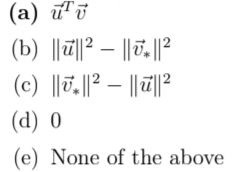A a B b C c D d E e
Engineering-Mathematics       Vectors
There are 45 questions to complete.